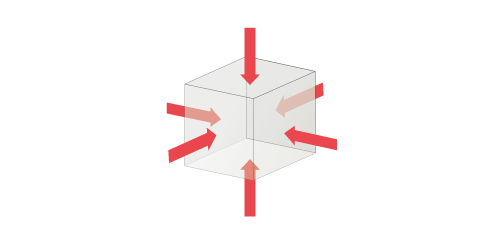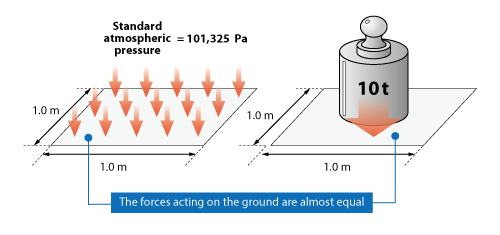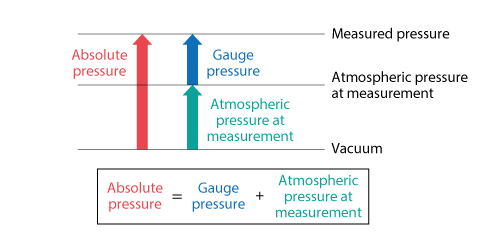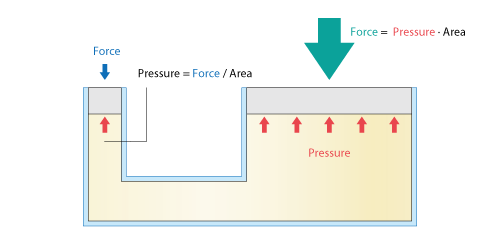# Want to Know More! Basics of Thermo-Fluid Analysis 13: Chapter 3 Flow 3.2.2 Pressure

#### 3.2.2 Pressure

Pressure is a force acting on an object per unit area and its unit is Pa.
Pressure is a scalar quantity and the product of pressure and the area of the face of an object is the normal force acting on the face. Pa is equivalent to N/m2 and therefore, the unit N is used for the force obtained by multiplying pressure and area.Figure3.9 Directions of pressure acting on surfaces

The standard atmospheric pressure on the ground is 101,325 Pa, which corresponds approximately to the weight of a 10 t object acting on a 1 m2 area.Figure 3.10 Pressure and force

There are two expression methods for pressure: absolute pressure and gauge pressure. Absolute pressure is zero-referenced against a perfect vacuum. The aforementioned standard atmospheric pressure is expressed with absolute pressure. On the other hand, gauge pressure is zero-referenced against ambient air pressure. Thus, if atmospheric pressure is different depending on a climate and a place, the two same gauge pressures may have different absolute pressure. Figure 3.11 shows the relationship between absolute pressure and gauge pressure. The pressure measured by most instruments is gauge pressure.Figure 3.11 Relationship between absolute pressure and gauge pressure

Want to know more  Units of pressure
Many units are used for pressure.

As for hPa used for a weather forecast (in Japan), a relation of 1 hPa = 100 Pa holds. In addition, as for mbar used for a weather forecast until the beginning of 1990s, a relation of 1 mbar = 10-3 bar and that of bar = 100 Pa hold.

The unit atm is defined based on the standard atmospheric pressure and a relation of 1 atm = 101,325 Pa holds.

The unit mmHg used for blood pressure is defined by the height of a column of mercury that balances with the pressure. A relation of 760 mmHg = 101,325 Pa holds. This indicates that the height of a column of mercury, 760 mm, balances with the standard atmospheric pressure. The unit Torr expresses the same value of mmHg and a relation of 1 Torr = 1 mmHg holds.

The unit mmAq is based on the height of water column is used instead of mercury. A relation of 1 mmAq = 9.80665 Pa holds.

Table 3.2 Standard atmospheric pressure per unit
Unit Value
Pa 101,325
hPa 1,013.25
bar 1.01325
mbar 1,013.25
atm 1.0
mmHg 760.0
Torr 760.0
mmAq 10,332.3

Want to know more  Pascal’s law
When a confined container is filled with static fluid and the pressure anywhere in the container increases, the pressure in the fluid evenly increases. This is called Pascal’s law.

A familiar example in which the law is used is a hydraulic jack shown in Figure 3.12. Let us focus on the fact that pressure is obtained by dividing force by area. When a face to extract force (right side) is larger than the face to which force is applied (left side), force can be increased by the area ratio. By using this, a human can lift a heavier object.Figure 3.12 Mechanism of hydraulic jack